Courses

# Differential Equations - 4

## 20 Questions MCQ Test Topic-wise Tests & Solved Examples for IIT JAM Mathematics | Differential Equations - 4

Description
This mock test of Differential Equations - 4 for Mathematics helps you for every Mathematics entrance exam. This contains 20 Multiple Choice Questions for Mathematics Differential Equations - 4 (mcq) to study with solutions a complete question bank. The solved questions answers in this Differential Equations - 4 quiz give you a good mix of easy questions and tough questions. Mathematics students definitely take this Differential Equations - 4 exercise for a better result in the exam. You can find other Differential Equations - 4 extra questions, long questions & short questions for Mathematics on EduRev as well by searching above.
QUESTION: 1

Solution:
QUESTION: 2

Solution:
QUESTION: 3

### For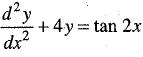solving by variation of parameters. The value of Wronskion W is

Solution:
QUESTION: 4

The initial value problem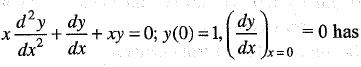Solution:
QUESTION: 5

Consider the following statements
I. A singular solution of differential equation satisfies the differential equation but is not a particular solution of the equation.
II. If T(x, y) = 0 is the equation of the tac-locus, then
T(x, y ) is a factor of the P-discriminant.
III. T(x, y ) is a factor of a c-discriminant.
IV. Cusp-locus has two distinct tangent.

Solution:
QUESTION: 6

The general solution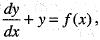where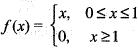andy(0) = 0 is

Solution:
QUESTION: 7

Which of the following pair of function are linear independent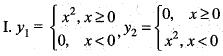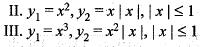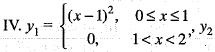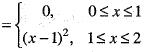Select the correct code

Solution:
QUESTION: 8

Singular solution of differential equation contains
I. arbitrary constant
II. can be obtained from general
III. do not contain arbitrary constant
IV. cannot be obtained from general solution

Solution:
QUESTION: 9

For non-homogeneous equation y' + p(x)y = r(x), if y1 and y2 are its solutions, then the solution of homogeneous equation y' + p(x)y = 0 is

Solution:
QUESTION: 10

The solution of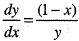represents

Solution:
QUESTION: 11

Consider the differential equation (x + y + 1) dx + (2x + 2y + 1)dy = 0. Which of the following statement is true?

Solution:

Here (x + y + 1)dx + (2x + 2y + 1)dy = 0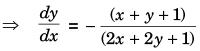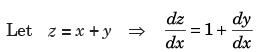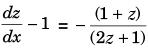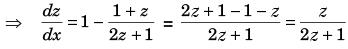By separables of variables,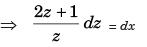Integrating (2z + log z) = x + c
⇒ 2(x + y) + log (x + y) = x + c.

QUESTION: 12

The orthogonal trajectory of the family x2 - y2 = C2 are given by

Solution:
QUESTION: 13

A. Singular solution contains no arbitrary constants.
B. Singular solution can be obtained from complete primitive.

Solution:
QUESTION: 14

Let the general solution of a differential equation be, y = aebx+c then order of the differential equation is,

Solution:
QUESTION: 15

If y1(x) = x and y2(x) = xex are two linearly independent solutions of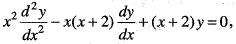then the interval on which they form a fundam ental set of solution is

Solution:
QUESTION: 16

Using the method of variation of parameters for the particular solution to the differential equation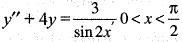Solution:
QUESTION: 17

The general solution of the system of differential equation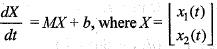and M a 2 x 2 matrixand a 2 x 1 constant vector b =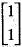is given by

Solution:
QUESTION: 18

For the ordinary differential equation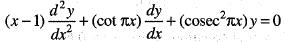which of the following statement is true?

Solution:
QUESTION: 19

If 2x(1 - y ) = K and g(x, y) = L are orthogonal families of curves where K and L constants, then g(x, y) is

Solution:
QUESTION: 20

If yand y2 are linearly independent solutions of the homogeneous equation L(y) =y"+p1(x)y' + p2(x)y = 0. Then, p1(x) and p2{x) are given by

Solution: